# Choosing a Classifier

July 21, 2015
By

Want to share your content on R-bloggers? click here if you have a blog, or here if you don't.

In order to illustrate the problem of chosing a classification model consider some simulated data,

```> n = 500
> set.seed(1)
> X = rnorm(n)
> ma = 10-(X+1.5)^2*2
> mb = -10+(X-1.5)^2*2
> M = cbind(ma,mb)
> set.seed(1)
> Z = sample(1:2,size=n,replace=TRUE)
> Y = ma*(Z==1)+mb*(Z==2)+rnorm(n)*5
> df = data.frame(Z=as.factor(Z),X,Y)```

A first strategy is to split the dataset in two parts, a training dataset, and a testing dataset.

```> df1 = training = df[1:300,]
> df2 = testing  = df[301:500,]```
• The Holdout Method: Training and Testing Datasets

The two datasets can be visualised below, with the training dataset on top, and the testing dataset below

```> plot(df1\$X,df1\$Y,pch=19,col=c(rgb(1,0,0,.4),
+ rgb(0,0,1,.4))[df1\$Z])```We can consider a simple classification tree.

```> library(rpart)
> fit = rpart(Z~X+Y,data=df1)
> pred = function(x,y) predict(fit,newdata=data.frame(X=x,Y=y))[,1]
```

To visualize it, use

```> vx=seq(-3,3,length=101)
> vy=seq(-25,25,length=101)
> z=matrix(NA,length(vx),length(vy))
> for(i in 1:length(vx)){
+   for(j in 1:length(vy))
+  {z[i,j]=pred(vx[i],vy[j])}}```

and

```> image(vx,vy,z,axes=FALSE,xlab="",ylab="")
> points(df1\$X,df1\$Y,pch=19,col=c(rgb(1,0,0,.4),
+ rgb(0,0,1,.4))[df1\$Z])```

We have on top the prediction on the training dataset, then below the prediction on the testing dataset. And finally on the bottom, the ROC curve for the testing dataset (black curve) as well as the training dataset (grey curve)

```> Y1=as.numeric(df1\$Z)-1
> Y2=as.numeric(df2\$Z)-1
> library(ROCR)
> S1 = predict(fit,newdata=df1)[,1]
> S2 = predict(fit,newdata=df2)[,1]
> pred <- prediction( S2, Y2 )
> perf <- performance( pred, "tpr", "fpr" )
> plot( perf )
> pred <- prediction( S1, Y1 )
> perf <- performance( pred, "tpr", "fpr" )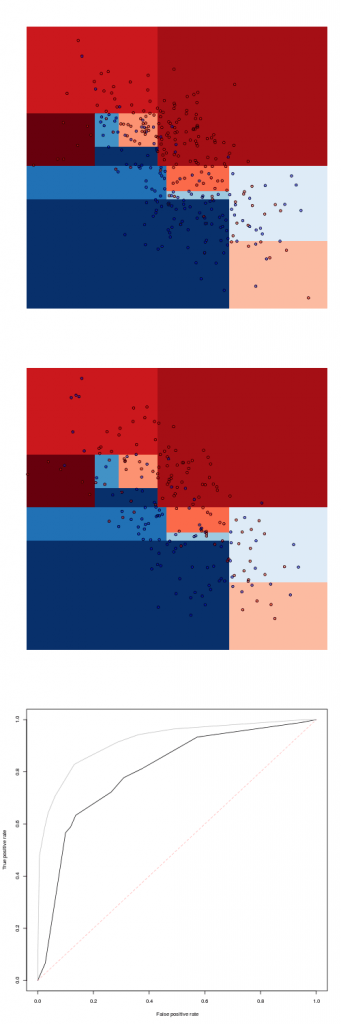From that tree, it is natural to get a prediction using a random forest

```> library(randomForest)
> fit=randomForest(Z~X+Y,data=df1)
```

To get a prediction, here, use

```> pred=function(x,y)
+ predict(fit,newdata=data.frame(X=x,Y=y),
+ type="prob")[,2]```Not that the fit is perfect on the training sample.  Another popoular model might be the logistic regression

```> fit=glm(Z~X+Y,data=df1,family=binomial)
> pred=function(x,y)
+ predict(fit,newdata=data.frame(X=x,Y=y),
+ type="response")```The later is very close to the linear discriminent analysis

```> library(MASS)
> fit=lda(Z~X+Y,data=df1,family=binomial)
> pred=function(x,y)
+ predict(fit,newdata=
+ data.frame(X=x,Y=y))\$posterior[,2]```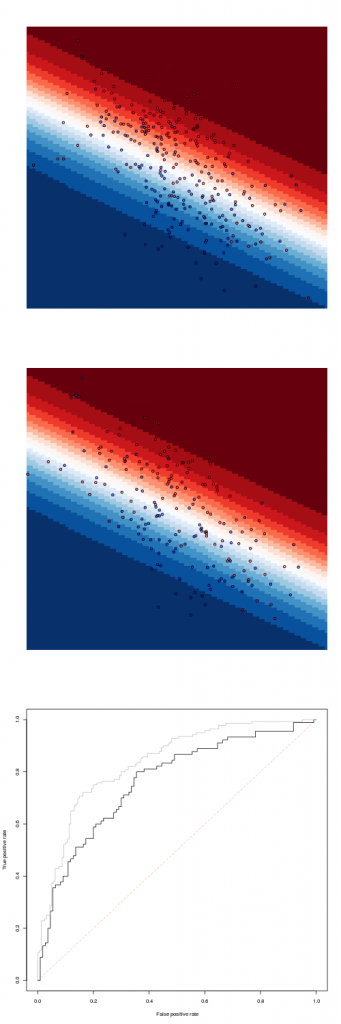```> fit=qda(Z~X+Y,data=df1,family=binomial)
> pred=function(x,y)
+ predict(fit,newdata=
+ data.frame(X=x,Y=y))\$posterior[,2]```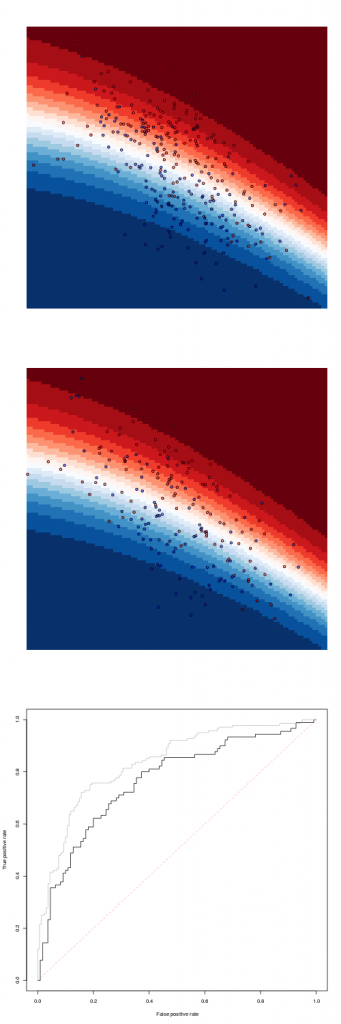To try very different models, consider a k-nearest neighbor
(here with 9 neighbors)

```> library(caret)
> fit=knn3(Z~X+Y,data=df1,k=9)
> pred=function(x,y)
+ predict(fit,newdata=data.frame(X=x,Y=y))[,2]```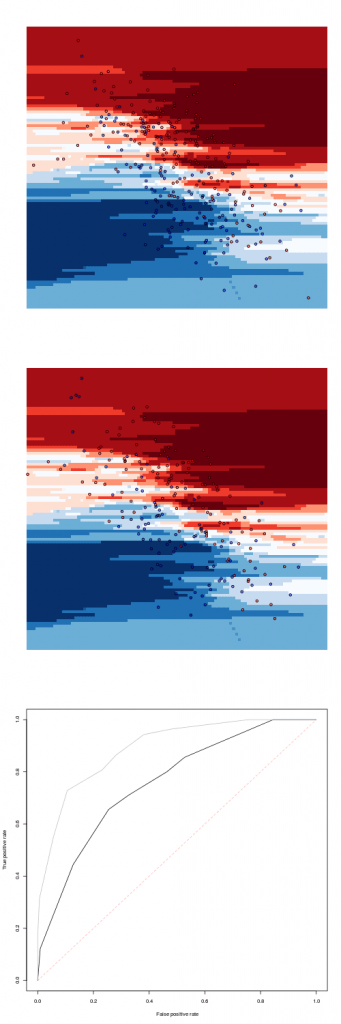some logistic regression with bivarriate splines

```> library(mgcv)
> fit=gam(Z~s(X,Y),data=df1,family=binomial)
> pred=function(x,y)
+ predict(fit,newdata=data.frame(X=x,Y=y),
+ type="response")```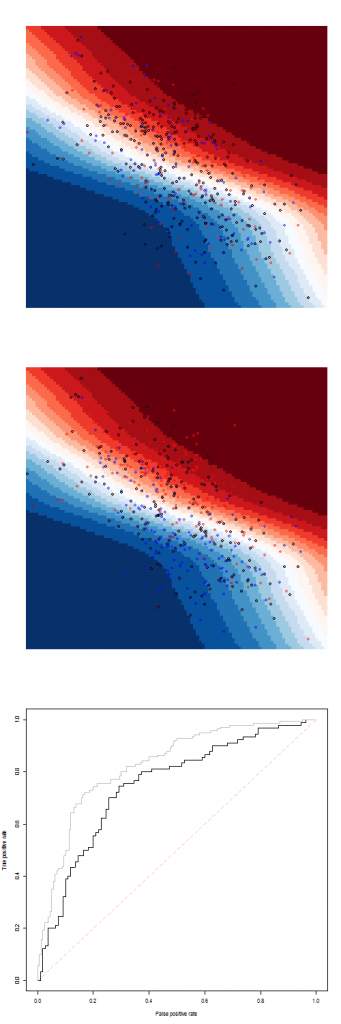```> library(dismo)
> df1\$Z01 = 1*(df1\$Z=="2")
> fit=gbm.step(data=df1, gbm.x = 2:3, gbm.y = 4,
+     family = "bernoulli", tree.complexity = 5,
+     learning.rate = 0.01, bag.fraction = 0.5)
> pred = function(x,y)
+ predict(fit,newdata=data.frame(X=x,Y=y),
+ type="response",n.trees=400)```Nevertheless, the use of this holdout method to create some training and testing datasets can be difficult. It can be done only when we have a lot of observations. And even so, we can still be unluckly when creating the testing sample (simply because of bad luck). An alternative is to use cross validation.

• Using Cross-Validation

Here, we consider one-leave-out cross-validation (but v-fold cross validation can also be consider, to get results faster).

Consider here a classification tree. To derive the cross validation ROC curve, for instance, use

```> FIT=list()
> for(i in 1:n)
+ FIT[[i]] = rpart(Z~X+Y,data=df[-i,])
> predict_i = function(i)
+ predict(FIT[[i]],newdata=df[i,])[,2]
> S = Vectorize(predict_i)(1:n)```

Here, I store the n models, where each time, one observation is removed, and then I get a prediction for the removed observation. And we can generate the ROC curve

```> Y = as.numeric(df\$Z)-1
> library(ROCR)
> pred = prediction( S, Y )
> perf = performance( pred, "tpr", "fpr" )
> plot( perf )```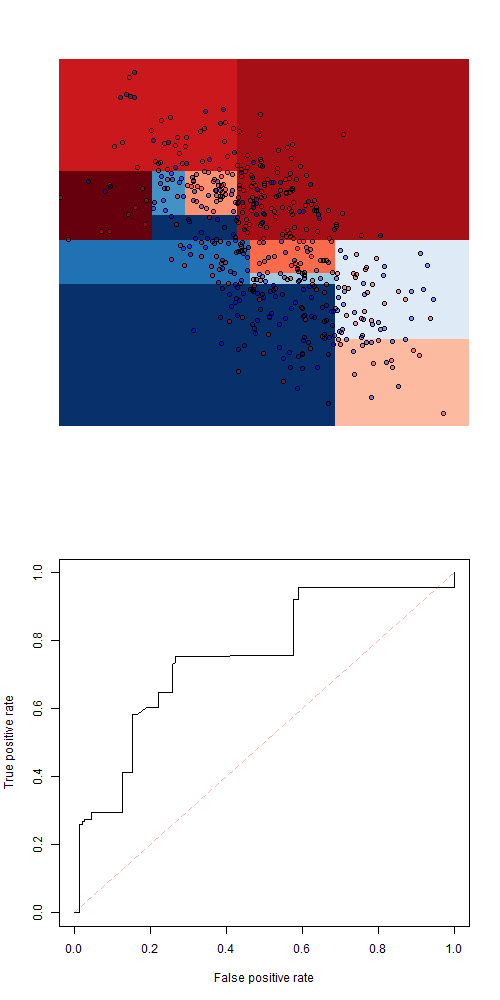Of course, one can easily get a code that runs faster, but that one is working on that small dataset.

If we consider a random forest, we get

```> FIT=list()
> for(i in 1:n)
+ FIT[[i]] = randomForest(Z~X+Y,data=df[-i,])
> predict_i = function(i)
+ predict(FIT[[i]],newdata=df[i,],
+ type="prob")[,2]
> S = Vectorize(predict_i)(1:n)```One can consider a logistic regression

```> FIT=list()
> for(i in 1:n)
+ FIT[[i]] = glm(Z~X+Y,data=df[-i,],
+ family=binomial)
> predict_i = function(i)
+ predict(FIT[[i]],newdata=df[i,],
+ type="response")
> S = Vectorize(predict_i)(1:n)```or a linear discriminent analysis

```> FIT=list()
> for(i in 1:n)
+ FIT[[i]] = lda(Z~X+Y,data=df[-i,],
+ family=binomial)
> predict_i = function(i)
+ predict(FIT[[i]],newdata=df[i,])\$posterior[,2]
> S = Vectorize(predict_i)(1:n)```while a quadratic discriminent analysis yields

```> FIT=list()
> for(i in 1:n)
+ FIT[[i]] =  qda(Z~X+Y,data=df[-i,],
+ family=binomial)
> predict_i = function(i)
+ predict(FIT[[i]],newdata=df[i,])\$posterior[,2]
> S = Vectorize(predict_i)(1:n)```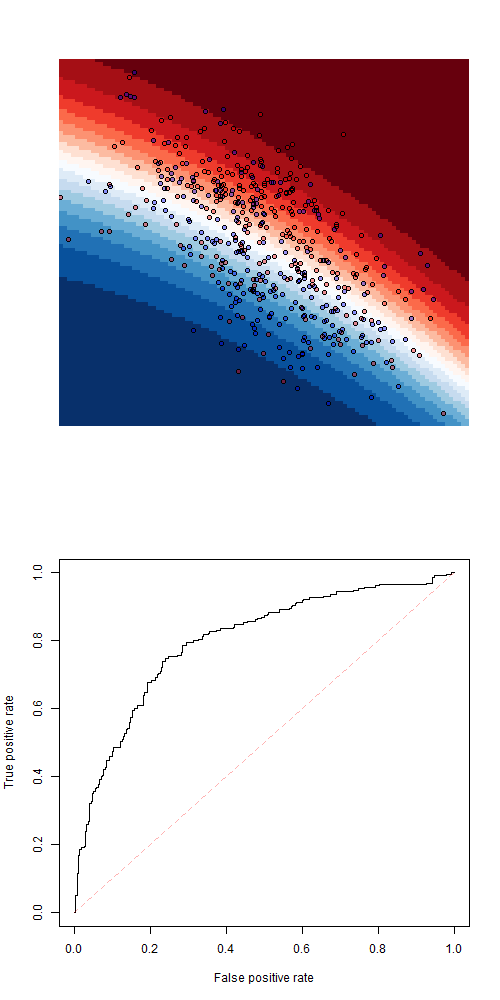Again, if we consider another type of model, such as a k-nearest neighbor (with k=5) we get

```> FIT=list()
> for(i in 1:n)
+ FIT[[i]] = knn3(Z~X+Y,data=df[-i,],k=5)
> predict_i = function(i)
+ predict(FIT[[i]],newdata=df[i,])[,2]
> S = Vectorize(predict_i)(1:n)```or a k nearest neighbor with k=9or k=21One can also consider a logistic regression with bivariate splinesor, last but not least, some gradient boosting model. On that one, we might have trouble storing 500 output from the gradient boosting function (the output is large). A faster code might be

```> VS = rep(NA,n)
> for(i in 1:n){
+  FIT = gbm.step(data=df[-i,],
+  gbm.x = 2:3, gbm.y = 4, family = "bernoulli",
+  tree.complexity = 5, learning.rate = 0.01,
+  bag.fraction = 0.5)
+  VS[i] = predict(FIT,newdata=df[i,],
+  n.trees=400)
+ }```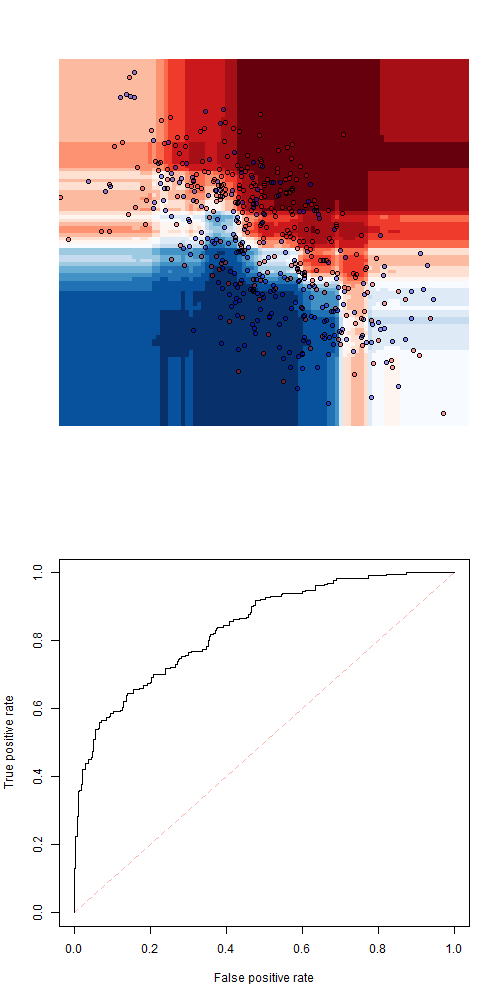Actually, it is now possible to compare all those models, with all ROC curves on a single graph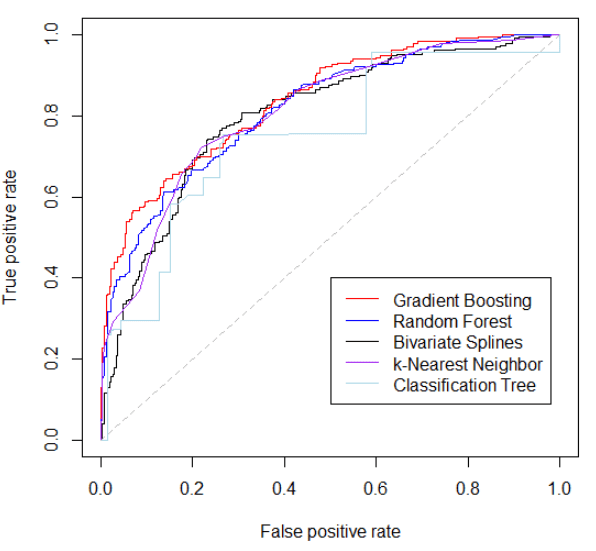R-bloggers.com offers daily e-mail updates about R news and tutorials about learning R and many other topics. Click here if you're looking to post or find an R/data-science job.
Want to share your content on R-bloggers? click here if you have a blog, or here if you don't.